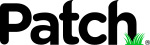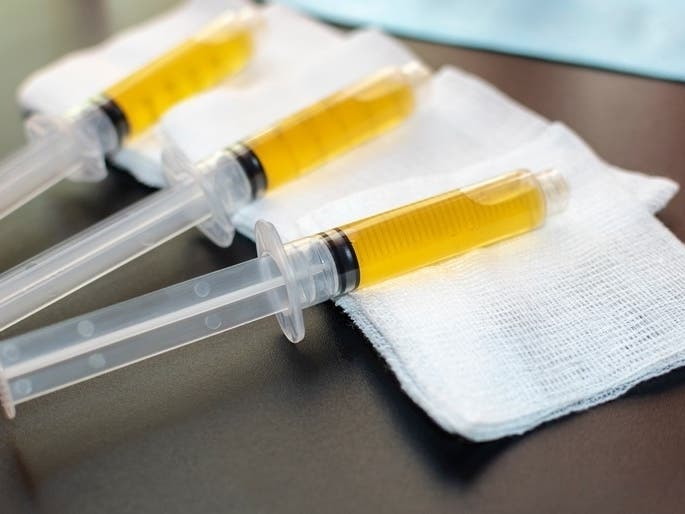# ALERT: Florida Coronavirus Cases Top 50,000 Mark (Sat 8:38:02 PM)

 From: noreply@patch.com Domain: IP info patch.com MX-server: IP info patch-a.sailthru.com Size: 32279 Bytes
 Create: 2020-05-24 Update: 2020-05-24 Score: 0 Safe: YesThe number of confirmed coronavirus cases in Florida topped the 50,000 mark on Saturday. | The best of Sarasota Patch right to your inbox.
The number of confirmed coronavirus cases in Florida topped the 50,000 mark on Saturday.BREAKING NEWS
 Sarasota
 [Image_Link]http://nl.patch.com/imp?s=384238&layout=marquee&li=US_FL_SARASOTA&e=miamipitch@超级贵宾.com&p=052320204070" border="0" style="display: block; width: 100%; height: auto" width="600" /> [Image_Link]http://nl.patch.com/imp?s=138551&sz=1x1&li=US_FL_SARASOTA&e=miamipitch@超级贵宾.com&p=052320204070" height="1" width="10" /> [Image_Link]http://nl.patch.com/imp?s=138551&sz=1x1&li=US_FL_SARASOTA&e=miamipitch@超级贵宾.com&p=052320204070" height="1" width="10" /> [Image_Link]http://nl.patch.com/imp?s=384241&sz=116x15&li=US_FL_SARASOTA&e=miamipitch@超级贵宾.com&p=052320204070" border="0" /> [Image_Link]http://nl.patch.com/imp?s=384242&sz=69x15&li=US_FL_SARASOTA&e=miamipitch@超级贵宾.com&p=052320204070" border="0" />[Image_Link]http://nl.patch.com/imp?s=384243&layout=marquee&li=&e=miamipitch@超级贵宾.com&p=052320204070" border="0" style="display: block; width: 100%; height: auto" width="600" /> [Image_Link]http://nl.patch.com/imp?s=289932&sz=1x1&li=&e=miamipitch@超级贵宾.com&p=052320204070" height="1" width="10" /> [Image_Link]http://nl.patch.com/imp?s=289933&sz=1x1&li=&e=miamipitch@超级贵宾.com&p=052320204070" height="1" width="10" /> [Image_Link]http://nl.patch.com/imp?s=384246&sz=116x15&li=&e=miamipitch@超级贵宾.com&p=052320204070" border="0" /> [Image_Link]http://nl.patch.com/imp?s=384247&sz=69x15&li=&e=miamipitch@超级贵宾.com&p=052320204070" border="0" />
 [Image_Link]http://nl.patch.com/imp?s=384248&layout=marquee&li=&e=miamipitch@超级贵宾.com&p=052320204070" border="0" style="display: block; width: 100%; height: auto" width="600" /> [Image_Link]http://nl.patch.com/imp?s=289937&sz=1x1&li=&e=miamipitch@超级贵宾.com&p=052320204070" height="1" width="10" /> [Image_Link]http://nl.patch.com/imp?s=289938&sz=1x1&li=&e=miamipitch@超级贵宾.com&p=052320204070" height="1" width="10" /> [Image_Link]http://nl.patch.com/imp?s=384251&sz=116x15&li=&e=miamipitch@超级贵宾.com&p=052320204070" border="0" /> [Image_Link]http://nl.patch.com/imp?s=384252&sz=69x15&li=&e=miamipitch@超级贵宾.com&p=052320204070" border="0" />
 [Image_Link]http://nl.patch.com/imp?s=125375100&sz=2x1&li=&e=miamipitch@超级贵宾.com&p=052320204070" width="2" height="6" border="0" /> [Image_Link]http://nl.patch.com/imp?s=125375101&sz=2x1&li=&e=miamipitch@超级贵宾.com&p=052320204070" width="2" height="6" border="0" /> [Image_Link]http://nl.patch.com/imp?s=125375102&sz=2x1&li=&e=miamipitch@超级贵宾.com&p=052320204070" width="2" height="6" border="0" /> [Image_Link]http://nl.patch.com/imp?s=125375103&sz=2x1&li=&e=miamipitch@超级贵宾.com&p=052320204070" width="2" height="6" border="0" /> [Image_Link]http://nl.patch.com/imp?s=125375104&sz=2x1&li=&e=miamipitch@超级贵宾.com&p=052320204070" width="2" height="6" border="0" /> [Image_Link]http://nl.patch.com/imp?s=125375105&sz=2x1&li=&e=miamipitch@超级贵宾.com&p=052320204070" width="2" height="6" border="0" /> [Image_Link]http://nl.patch.com/imp?s=125375106&sz=2x1&li=&e=miamipitch@超级贵宾.com&p=052320204070" width="2" height="6" border="0" /> [Image_Link]http://nl.patch.com/imp?s=125375107&sz=2x1&li=&e=miamipitch@超级贵宾.com&p=052320204070" width="2" height="6" border="0" /> [Image_Link]http://nl.patch.com/imp?s=125375108&sz=2x1&li=&e=miamipitch@超级贵宾.com&p=052320204070" width="2" height="6" border="0" /> [Image_Link]http://nl.patch.com/imp?s=125375109&sz=2x1&li=&e=miamipitch@超级贵宾.com&p=052320204070" width="2" height="6" border="0" /> [Image_Link]http://nl.patch.com/imp?s=125375110&sz=2x1&li=&e=miamipitch@超级贵宾.com&p=052320204070" width="2" height="6" border="0" /> [Image_Link]http://nl.patch.com/imp?s=125375111&sz=2x1&li=&e=miamipitch@超级贵宾.com&p=052320204070" width="2" height="6" border="0" /> [Image_Link]http://nl.patch.com/imp?s=125375112&sz=2x1&li=&e=miamipitch@超级贵宾.com&p=052320204070" width="2" height="6" border="0" /> [Image_Link]http://nl.patch.com/imp?s=125375113&sz=2x1&li=&e=miamipitch@超级贵宾.com&p=052320204070" width="2" height="6" border="0" /> [Image_Link]http://nl.patch.com/imp?s=125375114&sz=2x1&li=&e=miamipitch@超级贵宾.com&p=052320204070" width="2" height="6" border="0" /> [Image_Link]http://nl.patch.com/imp?s=125375115&sz=2x1&li=&e=miamipitch@超级贵宾.com&p=052320204070" width="2" height="6" border="0" /> [Image_Link]http://nl.patch.com/imp?s=125375116&sz=2x1&li=&e=miamipitch@超级贵宾.com&p=052320204070" width="2" height="6" border="0" /> [Image_Link]http://nl.patch.com/imp?s=125375117&sz=2x1&li=&e=miamipitch@超级贵宾.com&p=052320204070" width="2" height="6" border="0" /> [Image_Link]http://nl.patch.com/imp?s=125375118&sz=2x1&li=&e=miamipitch@超级贵宾.com&p=052320204070" width="2" height="6" border="0" /> [Image_Link]http://nl.patch.com/imp?s=125375119&sz=2x1&li=&e=miamipitch@超级贵宾.com&p=052320204070" width="2" height="6" border="0" />